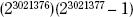NextPrevious

# What is a perfect number?

A perfect number is a number equal to the sum of all its proper divisors (divisors smaller than the number) including 1. The number 6 is the smallest perfect number; the sum of its divisors 1, 2, and 3 equals 6. The next three perfect numbers are 28, 496, and 8,126. No odd perfect numbers are known. The largest known perfect number is

It was discovered in 2001.Close

This is a web preview of the "The Handy Science Answer Book" app. Many features only work on your mobile device. If you like what you see, we hope you will consider buying. Get the App